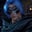Related Tags

pandas
python

# How to find the common rows between two DataFrames with merge()Gutha Vamsi Krishna

### Overview

DataFrame is a two-dimensional data structure in which data is aligned in rows and columns. In this Answer, we'll learn how to find the common rows between two DataFrames using the merge() function in Python pandas.

### Syntax

df1.merge(df2, how = 'inner' ,indicator=False)

In the syntax above, df1 and df2 are two DataFrames.

We pass inner as a value to the how parameter to get the common rows between two DataFrames. This operation is similar to InnerJoin in SQL.

### Example

import pandas as pd

#data frame 1
classA = pd.DataFrame(
{
"Student": ['John', 'Lexi', 'Augustin', 'Jane', 'Kate'],
"Age": [18, 17, 19, 17, 18]
}
)

#data frame 2
classB = pd.DataFrame(
{
"Student": ['John', 'Lexi', 'Bob', 'karl', 'Kate', 'Jane'],
"Age": [18, 17, 16, 19, 18, 20]
}
)

#get uncommon rows
print(classA.merge(classB, how = 'inner' ,indicator=False))
Merge common rows between two different DataFrames

### Explanation

In the above code snippet,

• Line 1: We import the pandas module, which contains methods to create DataFrames and modify them.
• Lines 4–8 and 13–19: We create two DataFrames that represent two classes of students. These data frames contain students' names and their ages.
• Line 21: We get the common students between the two classes classA and classB by merging both DataFrames using the merge() method and passing inner as the value to the how parameter.

RELATED TAGS

pandas
python

CONTRIBUTORGutha Vamsi Krishna